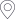﻿ 张国栋-烟台大学 数学与信息科学学院首页 > 师资力量 > 正文

## 张国栋

 姓名 张国栋 性别 男 出生年月 1986.08民族 汉 政治面貌 群众 职称/职务 教授 毕业学校 西安交通大学 学位 博士 专业 计算数学 研究兴趣 计算流体与物理，多物理场数值计算 通信地址 山东省烟台市莱山区清泉路30号 邮编 264005 联系电话 E-mail gdzhang@ytu.edu.cn  or  gdzhang2014@gmail.com 个 人 履 历（从大学填起） 时　间 单位 经　历 2004.9-2008.7 山东理工大学 信息与计算科学  理学学士 2008.9-2011.7 兰州大学 基础数学 理学硕士 2011.9-2015.12 西安交通大学 计算数学 理学博士 2014.9-2015.9 宾夕法尼亚州立大学 计算数学 联合培养博士 2016.2-至今 烟台大学 数学与信息科学学院 讲师，副教授 教 学 成 果 2018年指导孙盛宇等获“互联网+”大学生创新创业大赛优秀奖；2020年指导数162班孙飞鸿获烟台大学优秀本科学位论文；2022年指导周帅等获“互联网+”大学生创新创业大赛优秀奖；2023年指导陈建华等获山东省大学生科技创新大赛优秀奖；2023年指导李阳阳等获“挑战杯”大学生课外学术科技作品三等奖； 科 研 奖 励 2014年 博士生国家奖学金2018年 西安交通大学优秀博士论文2020年 美国数学会评论员 科 研 项 目 1.12171415，两相铁磁流体模型、算法、分析及模拟，国家自然科学基金面上项目，2022.01-2025.12，主持，在研2.12271468，变密度不可压缩两相流相场模型的高效数值方法及分析，国家自然科学基金面上项目，2023.01-2026.12，参与3/8,在研；3.11601468,磁流体方程的解耦算法及预处理快速求解方法，国家自然科学基金青年项目，2017.01-2019.12，主持, 结题4.11771375,非线性抛物方程有限体积元方法的后验误差估计及自适应算法,国家自然科学基金面上项目,2018.01-2021.12,参与3/10，结题；5.ZR2018MA008,奇异摄动问题的稳定化局部守恒数值方法及其在多孔介质流中的应用,山东省自然科学基金面上项目，2018.03-2021.6，参与4/7，结题 科 研 论 文 26.H. Su, G. D. Zhang(通讯), Energy stable schemes with second order temporal accuracy and decoupled structure for diffuse interface model of two-phase MHD，Communications in Nonlinear Science and Numerical Simulation，2023.25.G. D. Zhang, X. He, X. Yang, Reformulated weak formulation and efficient fully-discrete finite element method for a two-phase ferrohydrodynamics Shliomis model，SIAM Journal on Scientific Computing, 2023.24.G. D. Zhang, M. Yang, Y. He, Block preconditioners for energy stable schemes of MHD equations, Numerical Methods for Partial Differential Equations，2023.23.H. Su, G. D. Zhang(通讯), Highly Efficient and Energy Stable Schemes for the 2D/3D Diffuse Interface Model of Two-Phase Magnetohydrodynamics, Journal of Scientific Computing 2022.22.Y. He, G. D. Zhang, J. Zou, Fully Discrete Finite Element Approximation of the MHD Flow, Computational Methods in Applied Mathematics,2022.21.G. D. Zhang, X. He, X. Yang, A fully decoupled linearized finite element method with second-order temporal accuracy and unconditional energy stability for incompressible MHD equations, Journal of Computational Physics,202220.G. D. Zhang, X. Yang, Efficient and Stable Schemes for the Magnetohydrodynamic Potential Model, Communications in Computational Physics , 2021..19.G. D. Zhang, X. He, X. Yang, Decoupled, Linear, and UnconditionallyEnergy Stable Fully-Discrete Finite Element Numerical Scheme for A Two-Phase Ferrohydrodynamics Model, SIAM Journal on Scientific Computing 2021.18.K. Wang, G. D. Zhang(通讯), Unconditionally energy stable, splitting schemes for magneto-hydrodynamic equations, International Journal for Numerical Methods in Fluids, 2021.17.G. D. Zhang, C. Chen, Uniformly robust preconditioners for incompressible MHDsystem, Journal of Computational and Applied Mathematics, 2020.16.X. Yang, G. D. Zhang, Convergence Analysis for the Invariant Energy Quadratization (IEQ) Schemes for Solving the Cahn–Hilliard and Allen–Cahn Equations with General Nonlinear Potential, Journal of Scientific Computing 2020.15.G. D. Zhang, X. He, X. Yang, Fully decoupled, linear and unconditionally energy stable time discretization scheme for solving the magneto-hydrodynamic equations, Journal of Computational and Applied Mathematics,2020.14.G. D. Zhang, X. He, X. Yang, A Decoupled, Linear and Unconditionally Energy Stable Scheme with Finite Element Discretizations for Magneto-Hydrodynamic Equations, Journal of Scientific Computing 201913.X. Yang, G. D.Zhang(通讯), X. He, Convergence analysis of an unconditionally energy stable projection scheme for magneto-hydrodynamic equations, Applied Numerical Mathematics 2019.12.C. Chen, X. Zhang, G. D Zhang, Y. Zhang, A two-grid finite element method for nonlinear parabolic integro-differential equations, International Journal of Computer Mathematics,2019.11.G. D. Zhang, J. Yang, C. Bi, Second order unconditionally convergent and energy stable linearized scheme for MHD equations, Advances in Computational mathematics, 2018.10.J. Yang, Y. He, G. D. Zhang, On an efficient second order backward difference Newton scheme for MHD system.Journal of Mathematical analysis and applications, 2018.9.G. D. Zhang, Y. He, Decoupled schemes for unsteady MHD equations I:Time discretization, Numerical Methods for Partial Differential Equations, 2017.8.Y. Ma, J. Xu, G. D. Zhang, Error estimates for a structure preserving discretization of an incompressible MHD system, arXiv preprint arXiv:1608.03034, 20167.G. D. Zhang, Y. Zhang, Y. He, Two-Level Coupled and Decoupled Parallel Correction Methods for StationaryIncompressible Magnetohydrodynamics, Journal of Scientific Computing,2015.6.G. D. Zhang, Y. He, Unconditional convergence of the Euler semi-implicit scheme for the 3D incompressible MHD equations:numerical implementation, International Journal of Numerical Methods for Heat & Fluid Flow,20155.G. D. Zhang, X. Dong, Y. An, H. Liu, New conditions of stability and convergence of Stokes and Newton iterations for Navier-Stokes equations, Applied Mathematics and Mechanics, 2015.4.G. D. Zhang, Y. He, Decoupled schemes for unsteady MHD equations II:Finite element spatial discretization and numerical implementation, Computers & Mathematics with Applications,2015.3.G. D. Zhang, Y. He, D. Yang, Analysis of coupling iterations based on the finite element method for stationary magnetohydrodynamics on ageneral domain, Computers &Mathematics with Applications, 2014.2.G. D. Zhang, Y. He, Y. Zhang, Streamline diffusion finite element method for stationary incompressible magnetohydrodynamics, Numerical Methods for Partial Differential Equations, 2014.1.G. D. Zhang, H.-R.Sun, Multiple solutions for a fourth-order difference boundary value problem with parameter via variational approach, Applied Mathematical Modelling, 2012. 指 导 研 究 生 情 况 2022级计算数学专业，陈建华2021级计算数学专业, 周帅2018级计算数学专业，王琨，磁流体模型的解耦算法， 2021年毕业去向德州市人事单位。